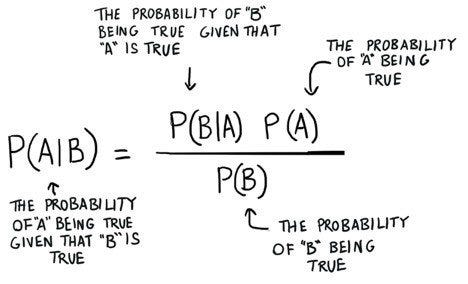# Subek's Blog# Understanding Naive Bayes for Natural Language Processing (NLP)

## Introduction

Machine learning algorithms play a crucial role in the field of natural language processing (NLP). Naive Bayes is one of the popular and effective algorithms used in NLP. In this article, we will explore the fundamentals of Naive Bayes and its applications in various NLP tasks, highlighting its simplicity, efficiency, and effectiveness.

## What is Naive Bayes?

Naive Bayes is a probabilistic machine learning algorithm based on Bayes' theorem. The term "naive" indicates the assumption of independence among the features. Despite this assumption, Naive Bayes has proven to be remarkably effective in many NLP tasks, such as sentiment analysis.

## Bayes' Theorem:

Before diving into Naive Bayes, let's first understand the underlying principle of Bayes' theorem. It provides a way to update probabilities based on new evidence and is expressed as:

P(A|B) = P(B|A) * P(A) / P(B)

Where:

P(A|B): Probability of event A occurring given event B has occurred.

P(B|A): Probability of event B occurring given that event A has occurred.

P(A): Prior probability of event A.

P(B): Prior probability of event B.## Naive Bayes for NLP:

Naive Bayes finds application in various NLP use cases, including sentiment analysis, spam detection, document classification, and more. It leverages Bayes' theorem to calculate the probability of a given text belonging to a particular class, such as positive or negative sentiment. It is based on the frequencies of words or features in the given text.

## Training and Classification:

Like other machine learning algorithms, Naive Bayes undergoes a training phase. During this phase, it learns the statistical properties of the text data by calculating the probabilities of different words or features occurring in each class. This information is then used to make predictions on new and unseen data.

## Naive Bayes Assumption:

An assumption made in the implementation of Naive Bayes is called the independence assumption. It assumes that the presence or absence of a particular feature (e.g., a word) is independent of the presence or absence of other features. Although this assumption may not hold true for all NLP tasks, Naive Bayes can still yield good results in practice due to its simplicity and efficiency.

## Laplace Smoothing:

Dealing with words that were not present in the training data is a common challenge in NLP. To address this issue, we can employ Laplace smoothing. It adds a small value to the word count, ensuring that no probability estimate is zero. Thus, we avoid getting null probabilities for unseen words.

## Pros and Cons of Naive Bayes in NLP:

### Pros:

• Simple and efficient to implement.

• Performs well with high-dimensional data, such as text.

• Can be trained with little training data.

### Cons:

• Sensitive to irrelevant features.

• Struggles with rare or unseen words, despite Laplace smoothing.

• The independence assumption might not always hold for certain NLP tasks.